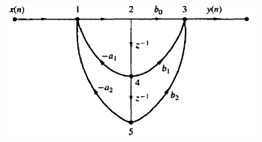Test: IIR System Structures

# Test: IIR System Structures

Test Description

## 10 Questions MCQ Test Signals and Systems | Test: IIR System Structures

Test: IIR System Structures for Electrical Engineering (EE) 2023 is part of Signals and Systems preparation. The Test: IIR System Structures questions and answers have been prepared according to the Electrical Engineering (EE) exam syllabus.The Test: IIR System Structures MCQs are made for Electrical Engineering (EE) 2023 Exam. Find important definitions, questions, notes, meanings, examples, exercises, MCQs and online tests for Test: IIR System Structures below.
Solutions of Test: IIR System Structures questions in English are available as part of our Signals and Systems for Electrical Engineering (EE) & Test: IIR System Structures solutions in Hindi for Signals and Systems course. Download more important topics, notes, lectures and mock test series for Electrical Engineering (EE) Exam by signing up for free. Attempt Test: IIR System Structures | 10 questions in 10 minutes | Mock test for Electrical Engineering (EE) preparation | Free important questions MCQ to study Signals and Systems for Electrical Engineering (EE) Exam | Download free PDF with solutions
 1 Crore+ students have signed up on EduRev. Have you?
Test: IIR System Structures - Question 1

### If M and N are the orders of numerator and denominator of rational system function respectively, then how many multiplications are required in direct form-I realization of that IIR filter?

Detailed Solution for Test: IIR System Structures - Question 1

Explanation: From the direct form-I realization of the IIR filter, if M and N are the orders of numerator and denominator of rational system function respectively, then M+N+1 multiplications are required.

Test: IIR System Structures - Question 2

### If M and N are the orders of numerator and denominator of rational system function respectively, then how many additions are required in direct form-I realization of that IIR filter?

Detailed Solution for Test: IIR System Structures - Question 2

Explanation: From the direct form-I realization of the IIR filter, if M and N are the orders of numerator and denominator of rational system function respectively, then M+N additions are required.

Test: IIR System Structures - Question 3

### If M and N are the orders of numerator and denominator of rational system function respectively, then how many memory locations are required in direct form-I realization of that IIR filter?

Detailed Solution for Test: IIR System Structures - Question 3

Explanation: From the direct form-I realization of the IIR filter, if M and N are the orders of numerator and denominator of rational system function respectively, then M+N+1 memory locations are required.

Test: IIR System Structures - Question 4

In direct form-I realization, all-pole system is placed before the all-zero system.

Detailed Solution for Test: IIR System Structures - Question 4

Explanation: In direct form-I realization, all-zero system is placed before the all-pole system.

Test: IIR System Structures - Question 5

If M and N are the orders of numerator and denominator of rational system function respectively, then how many memory locations are required in direct form-II realization of that IIR filter?

Detailed Solution for Test: IIR System Structures - Question 5

Explanation: From the direct form-II realization of the IIR filter, if M and N are the orders of numerator and denominator of rational system function respectively, then Max[M,N] memory locations are required.

Test: IIR System Structures - Question 6

The basic elements of a flow graph are branches and nodes.

Detailed Solution for Test: IIR System Structures - Question 6

Explanation: A signal flow graph provides an alternative, but an equivalent graphical representation to a block diagram structure that we have been using to illustrate various system realization. The basic elements of a flow graph are branches and nodes.

Test: IIR System Structures - Question 7

Which of the following is true for the given signal flow graph?Detailed Solution for Test: IIR System Structures - Question 7

Explanation: The equivalent filter structure of the given signal flow graph in the direct form-II is given by as
Thus from the above structure, the system has two zeros and two poles.

Test: IIR System Structures - Question 8

What are the nodes that replace the adders in the signal flow graphs?

Detailed Solution for Test: IIR System Structures - Question 8

Explanation: Summing node is the node which is used in the signal flow graph which replaces the adder in the structure of a filter.

Test: IIR System Structures - Question 9

The output signal of a system is extracted at a sink node.

Detailed Solution for Test: IIR System Structures - Question 9

Explanation: The input to a system originates at a source node and the output signal is extracted at a sink node.

Test: IIR System Structures - Question 10

If we reverse the directions of all branch transmittances and interchange the input and output in the flow graph, then the resulting structure is called as:

Detailed Solution for Test: IIR System Structures - Question 10

Explanation: According to the transposition or flow-graph reversal theorem, if we reverse the directions of all branch transmittances and interchange the input and output in the flow graph, then the system remains unchanged. The resulting structure is known as transposed structure or transposed form.

## Signals and Systems

32 videos|76 docs|63 tests
 Use Code STAYHOME200 and get INR 200 additional OFF Use Coupon Code
Information about Test: IIR System Structures Page
In this test you can find the Exam questions for Test: IIR System Structures solved & explained in the simplest way possible. Besides giving Questions and answers for Test: IIR System Structures, EduRev gives you an ample number of Online tests for practice

## Signals and Systems

32 videos|76 docs|63 tests